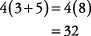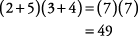## Grouping Symbols and Order of Operations

Three common types of grouping symbols—parentheses ( ), brackets [ ], and braces { }—are used to group numbers or variables (letters). The most commonly used grouping symbols are parentheses. Operations inside parentheses can be done before any other operations in order to simplify the problem.

##### Example 1

Simplify 4(3 + 5).##### Example 2

Simplify (2 + 5)(3 + 4).Brackets and braces are less commonly used grouping symbols and should be used after parentheses. Parentheses are to be used first, then brackets, and then braces: { [ ( ) ] }. Sometimes, instead of brackets or braces, you will see the use of larger parentheses.

##### Example 3

Simplify ((2+3) × 4) + 1.An expression using all three grouping symbols looks like this:

2{1 + [4(2+1) + 3]}

##### Example 4

Simplify 2{1 + [4(2+1) + 3]}.

Notice that you work from the inside out.